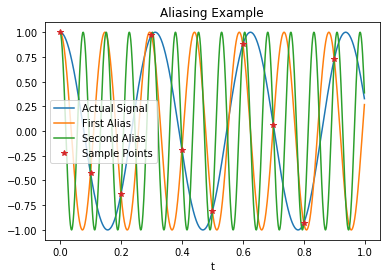# Aliasing and the Nyquist Theorem¶

Aliasing can be seen when signals are at the $nf_s \pm f_0$ where $f_s$ is the sampling frequency, and $f_0$ is the frequency in the baseband range, $-f_s/2 < f_0 < f_s/2$ and $n$ is any integer.

In :
import matplotlib.pyplot as plt
import numpy as np
from IPython.display import Latex

N = 10
T = 0.1
f_s = 1/T
T_0 = N*T
f_0 = 3.2  # Harmonic number.
display(Latex('The sampling frequency, $f_s$ in Hz is:'), f_s,)
print('The Nyquist frequency is ', 1/2/T, 'Hz.')
print('The actual signal frequency is ', f_0, 'Hz.')
k = np.arange(0,N)
t = np.arange(0, T_0, 0.01*T)
kt = k*T
plt.plot(t, np.cos(2*np.pi*f_0*t), t, np.cos(2*np.pi*(-f_0 + 1/T)*t),
t, np.cos(2*np.pi*(f_0 + 1/T)*t), kt, np.cos(2*np.pi*f_0*kt),'*')
plt.xlabel('t')
plt.title('Aliasing Example')
plt.legend(['Actual Signal', 'First Alias', 'Second Alias', 'Sample Points'])
plt.show()

The sampling frequency, $f_s$ in Hz is:
10.0
The Nyquist frequency is  5.0 Hz.
The actual signal frequency is  3.2 Hz.In [ ]: